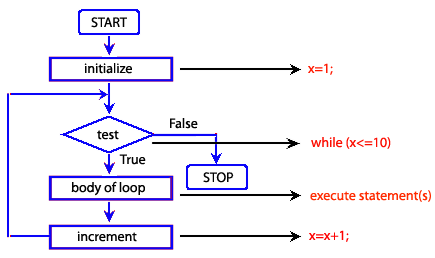JavaScript while loop

Description

In JavaScript the while loop is simple, it executes its statements repeatedly as long as the condition is true. The condition is checked every time at the beginning of the loop.

Syntax

while (condition)
{
statements
}

Pictorial Presentation:Example:

The following web document calculates the sum of odd numbers between 0 to 10. The while loop starts with x = 0 and runs until it equals to 10. If the remainder of x/2 is not equals to 0 we add x with y and after completion of the loop y return the sum of odd numbers.

HTML Code

<!DOCTYPE html>
<meta charset=utf-8>
<title>JavaScript while statement :  Example-1</title>
<body> <body> <h1 style="color: red">JavaScript : while statement</h1> <h3> The while loop calculate the sum of odd numbers between 0 to 10. </h3> <p id="result">List of numbers :</p> <script src="while-statement-example1.js"></script> </body> </html>

JS Code

var x = 1;
var y = 0;
var z = 0;
document.getElementById("result").innerHTML = "List of numbers : ";
while (x <=10 )
{
z = x %  2;
if (z !== 0)
{
var newParagraph1 = document.createElement("p");
var newText1 = document.createTextNode(x);
newParagraph1.appendChild(newText1);
document.body.appendChild(newParagraph1);
y=y+x;
}
x++;
}
var newParagraph1 = document.createElement("p");
var newText1 = document.createTextNode("The sum of even numbers between 0 to 10 is : " + y);
newParagraph1.appendChild(newText1);
document.body.appendChild(newParagraph1);

View the example in the browser

Practice the example online

See the Pen while-1 by w3resource (@w3resource) on CodePen.

Previous: JavaScript do while loop
Next: JavaScript for loop

﻿